# NCERT Exemplar Class 11 Physics Solutions for Chapter 13 - Kinetic Theory

NCERT Exemplar Solutions for Class 11 Physics Chapter 13 Kinetic Theory provide answers to the questions given in the NCERT Exemplar book. The extra questions provided here are prepared by the subject experts at BYJU’S, which will assist you in preparation for CBSE Class 11 and entrance exams like NEET, JEE Main and Advanced, AIEEE, etc. This NCERT Exemplar Class 11 Physics Chapter 13 PDF contains MCQs, match the following, fill in the blanks and true or false type of questions along with numerical problems, important derivations, worksheets, exercises and HOTS. The kinetic theory is a theory which applies to different particles, such as atoms and molecules. Kinetic theory is usually applied to the properties of gases. The Brownian motion gives a pretty good visualisation of the flow of particles and the collisions that may occur.

## Download the PDF of NCERT Exemplar Solutions for Class 11 Physics Chapter 13 Kinetic Theory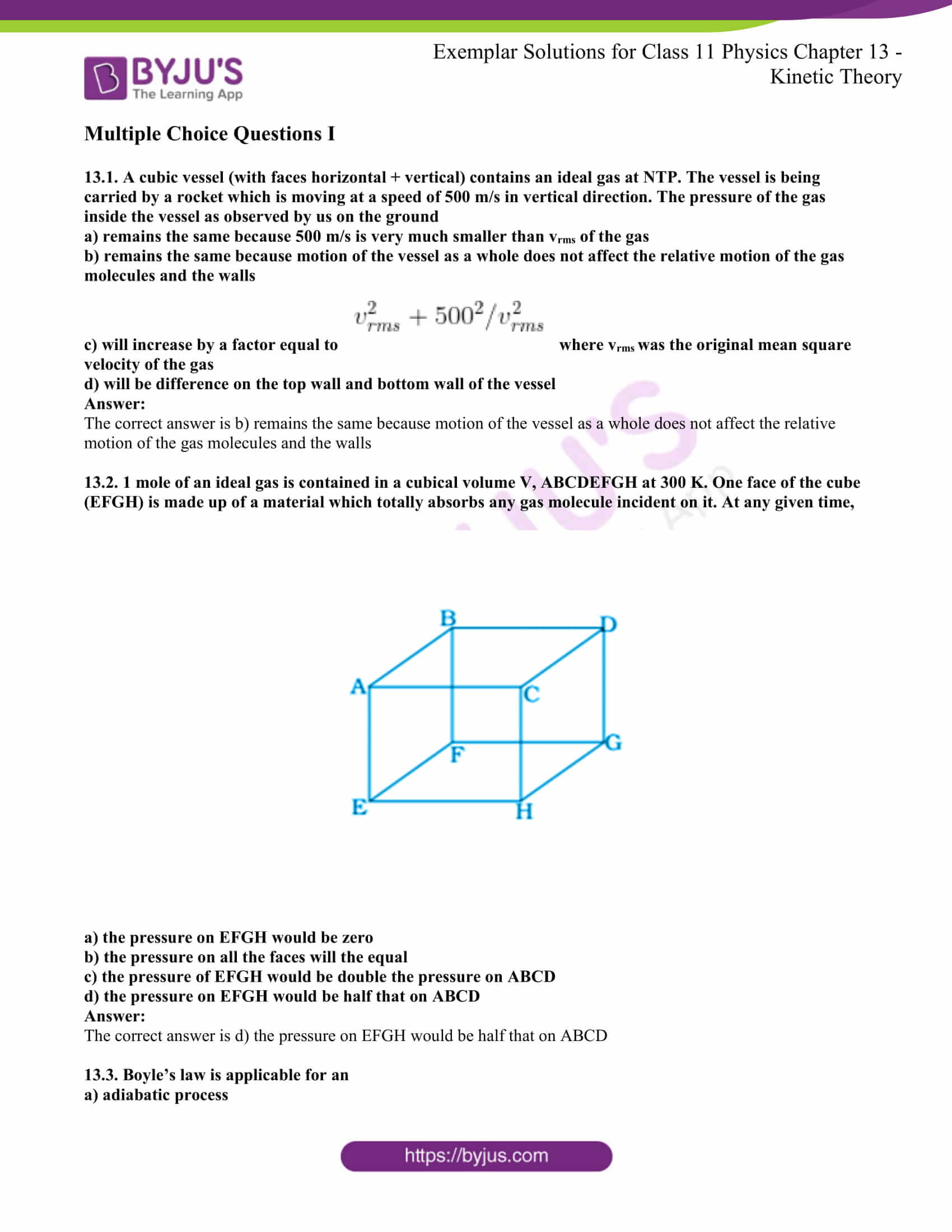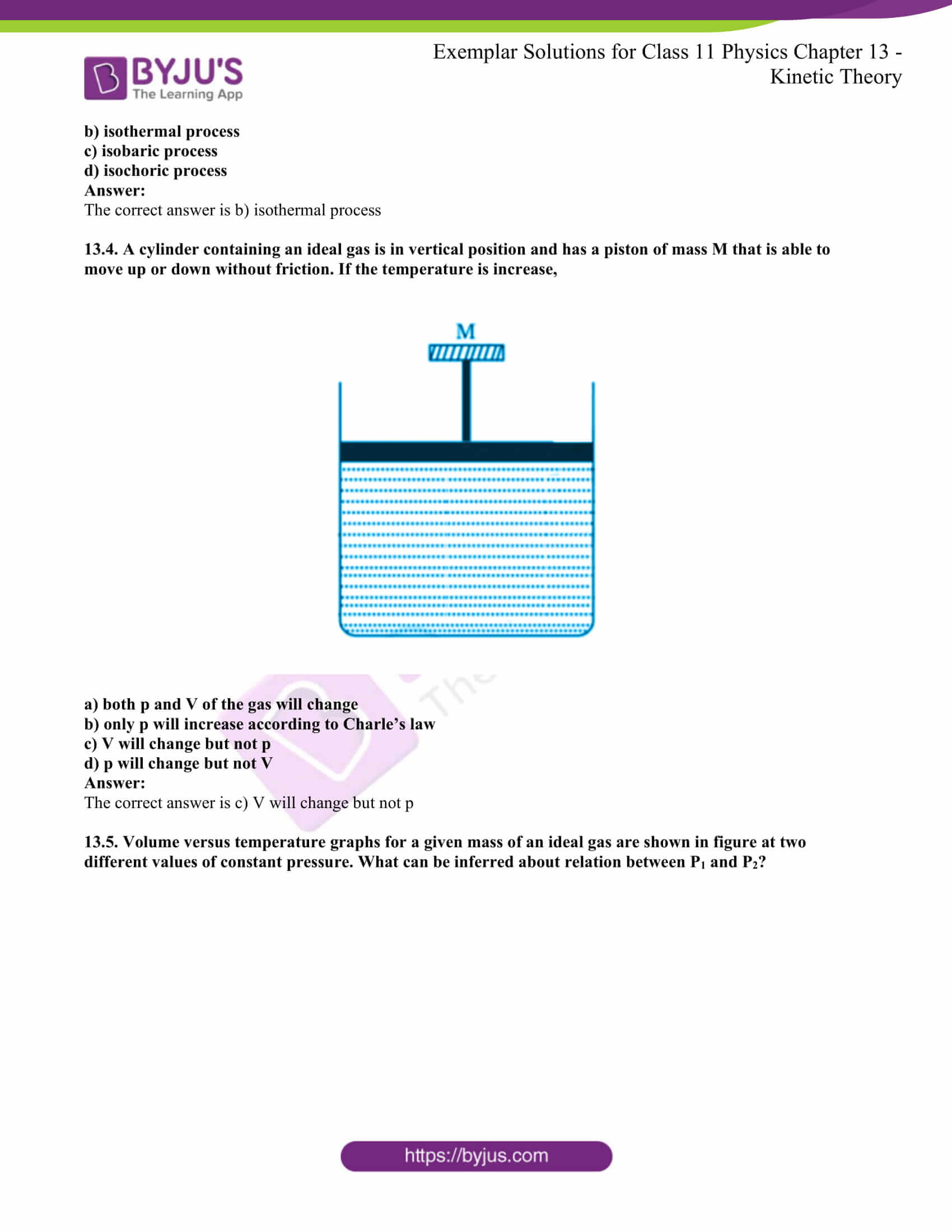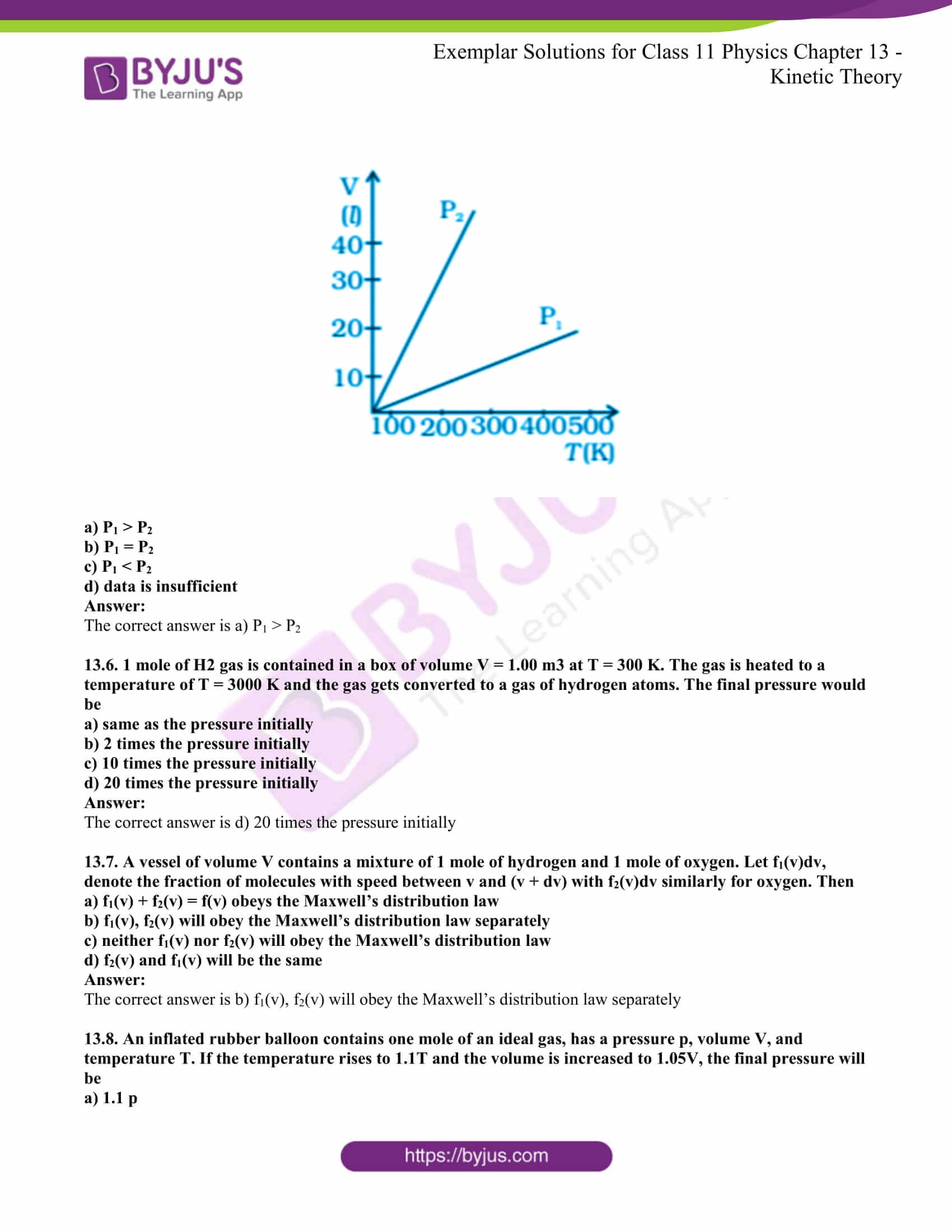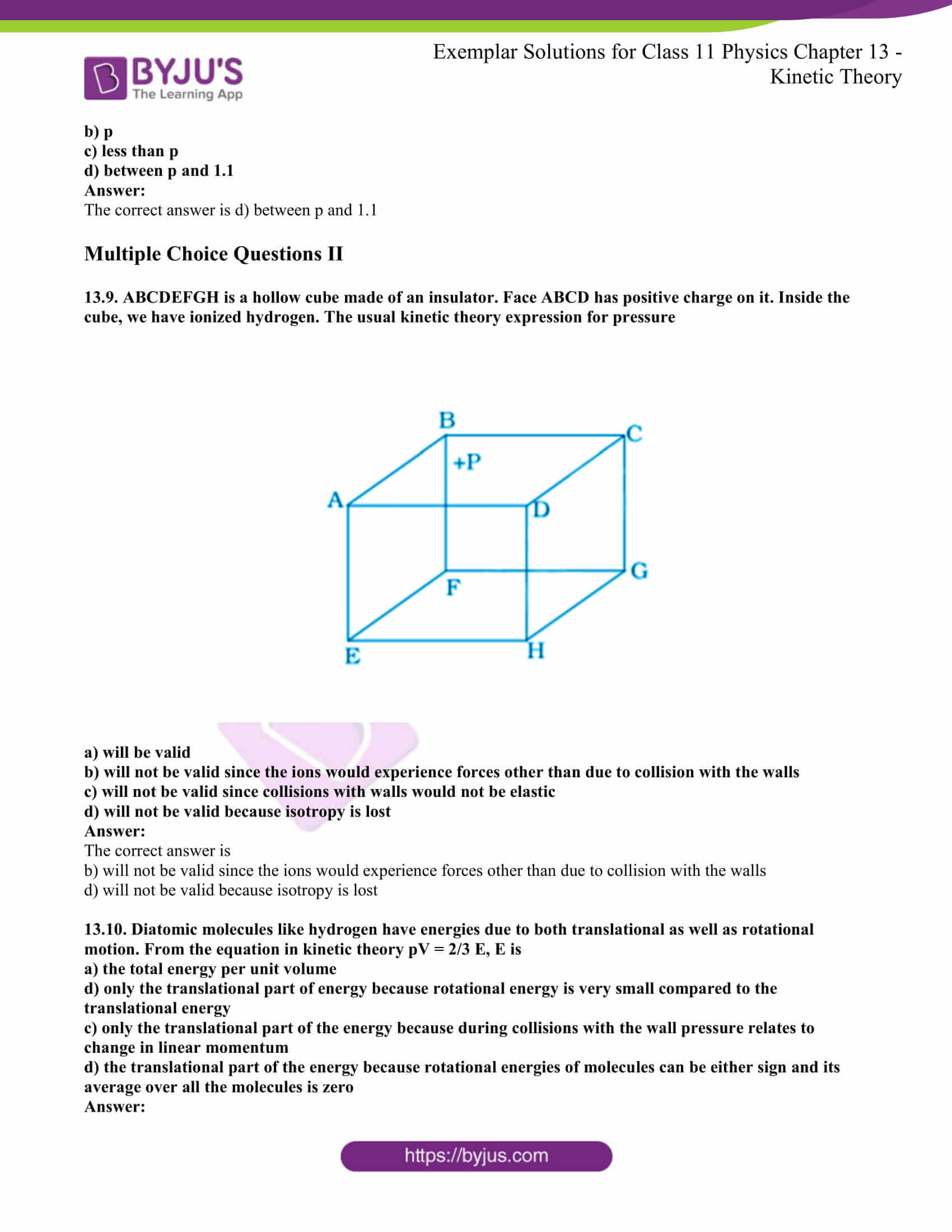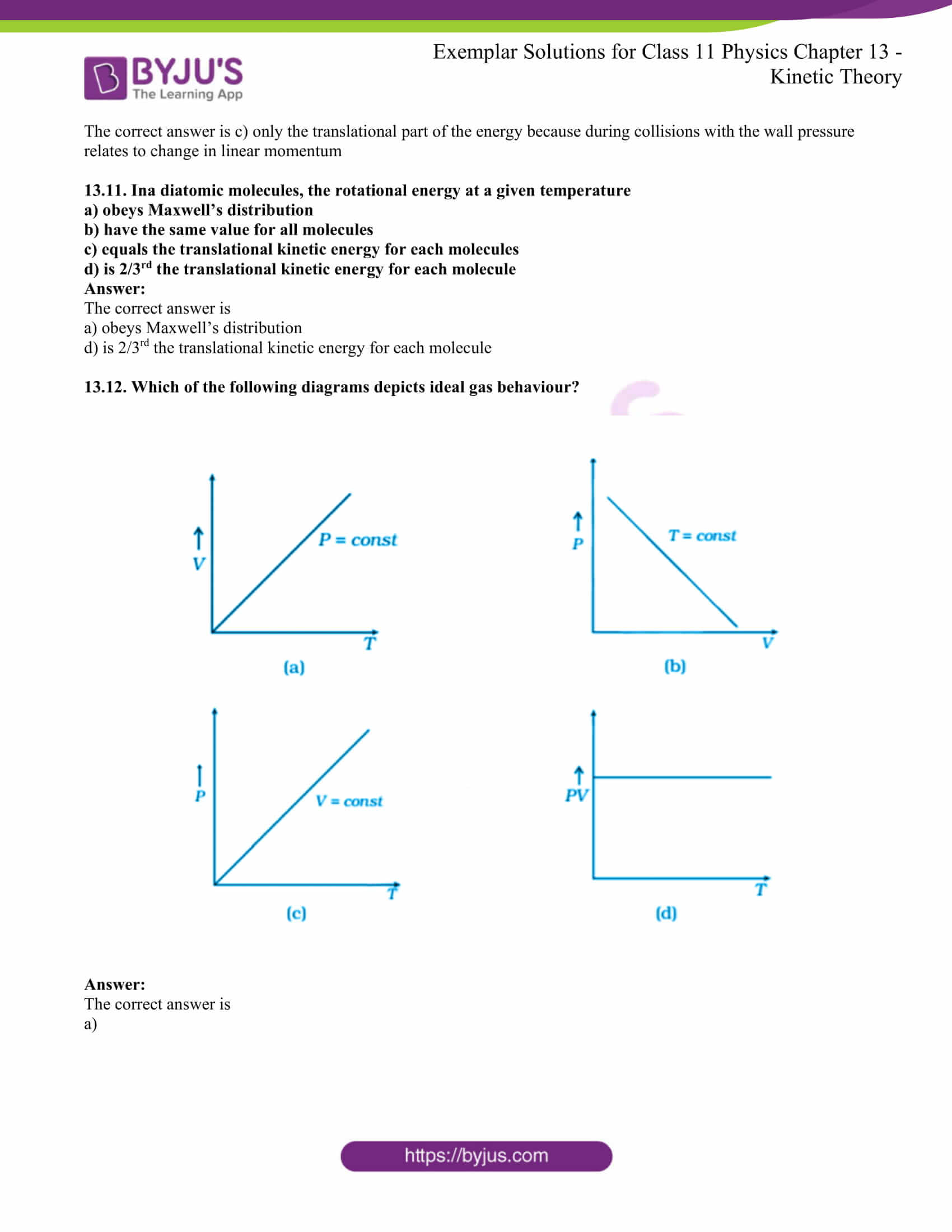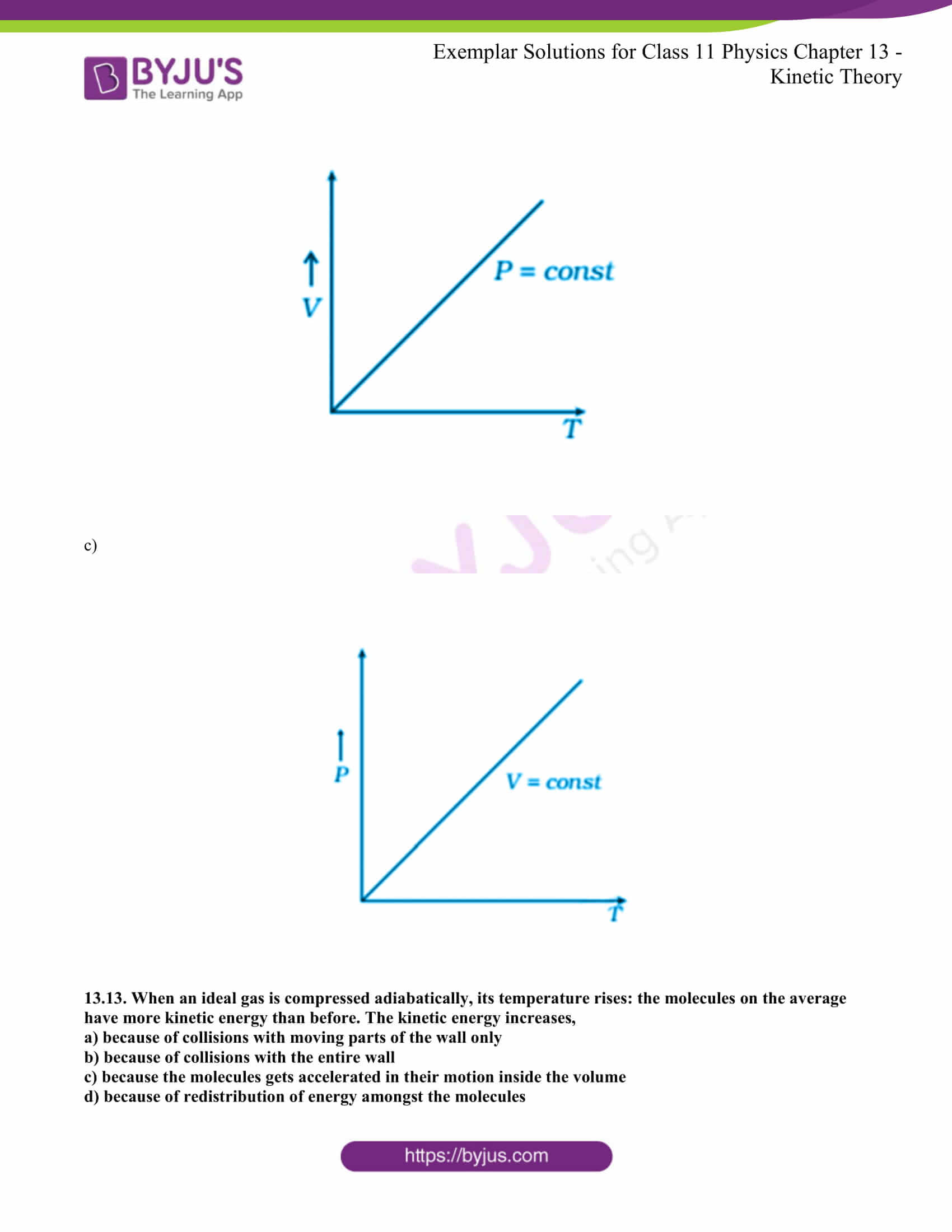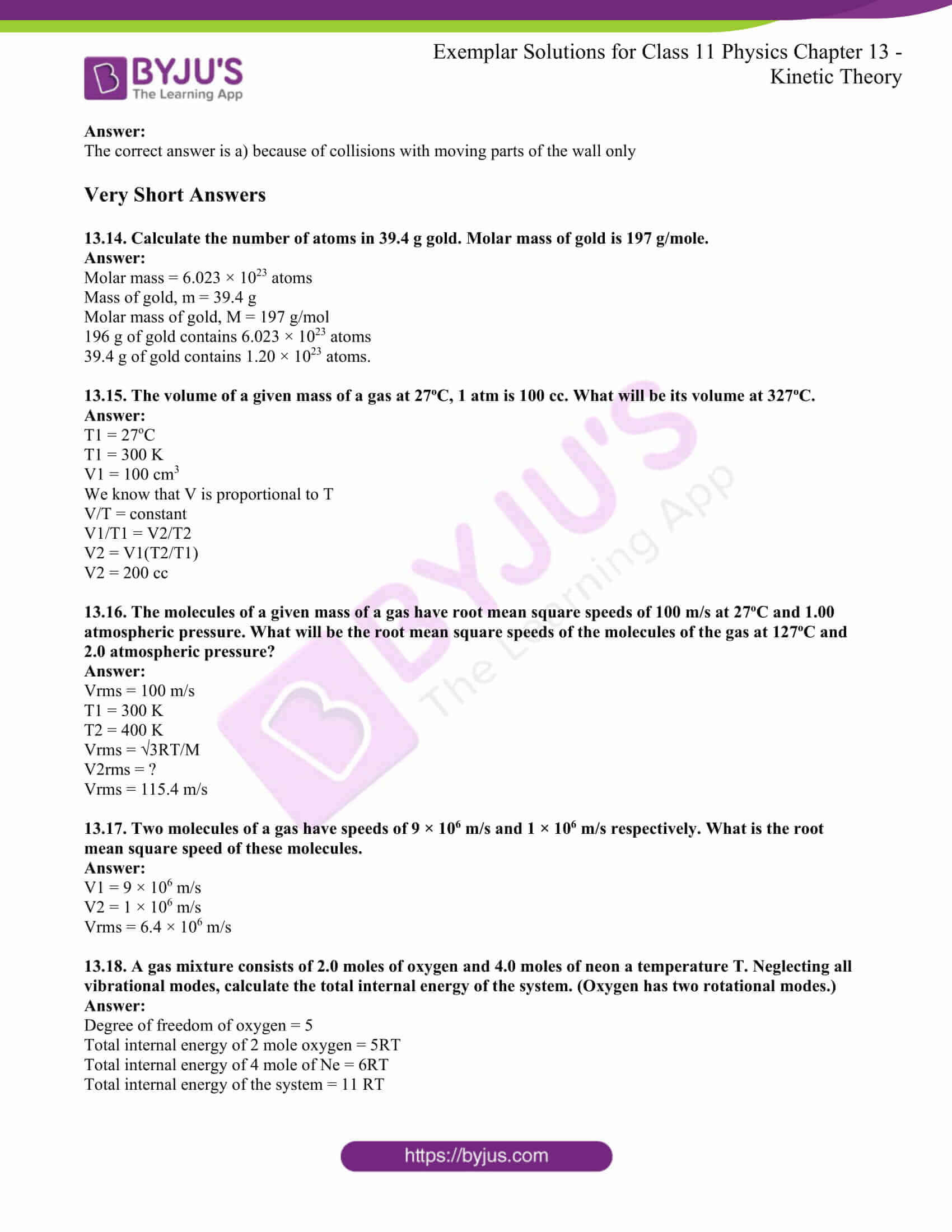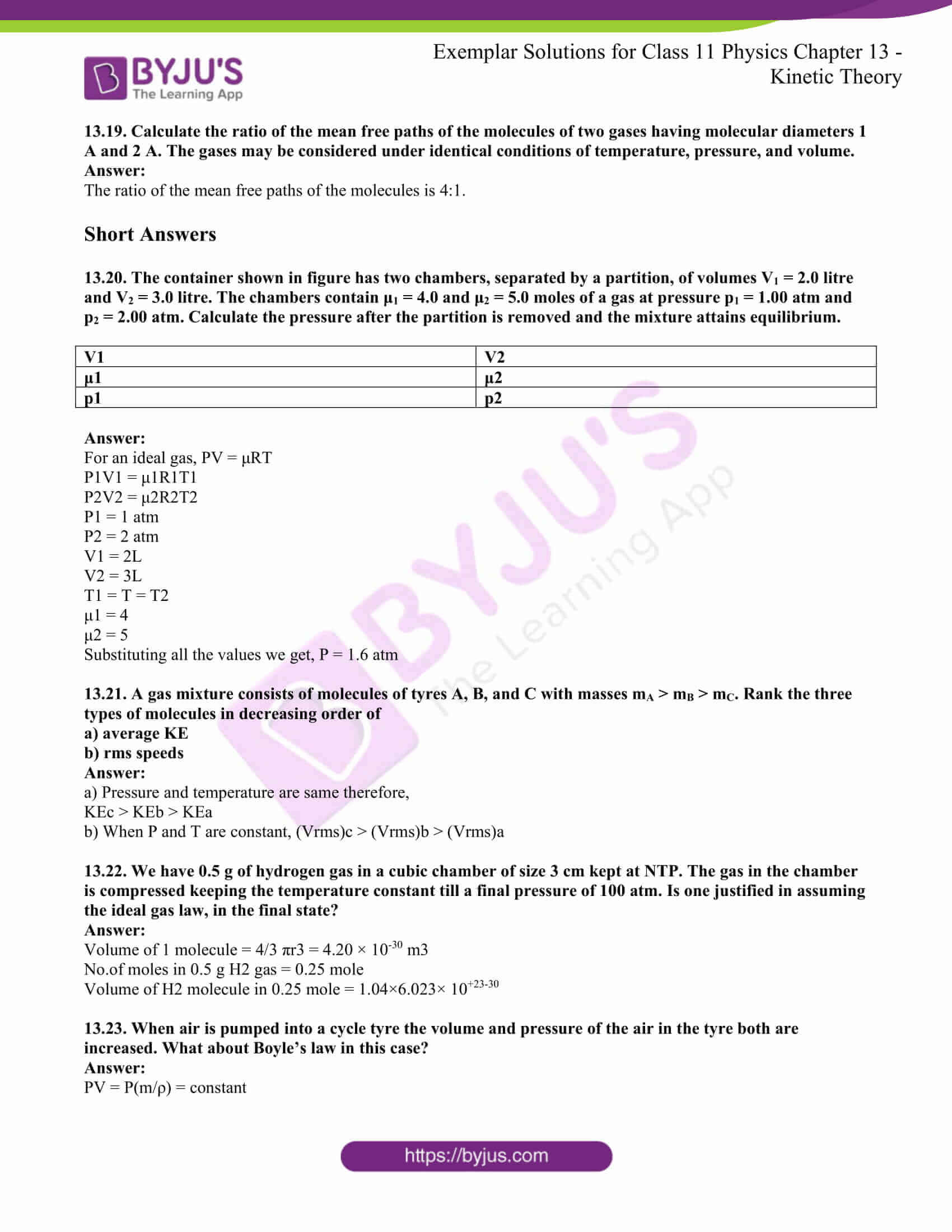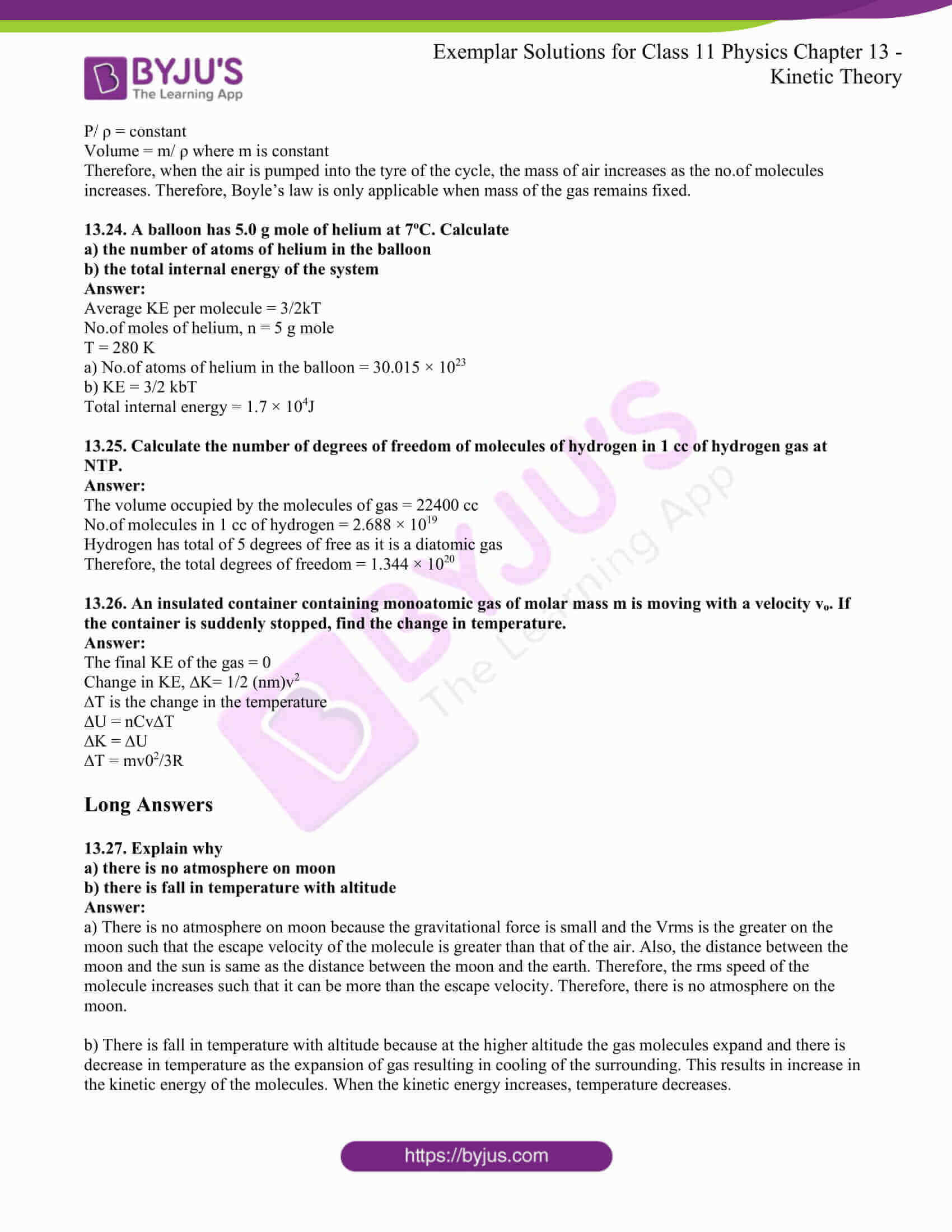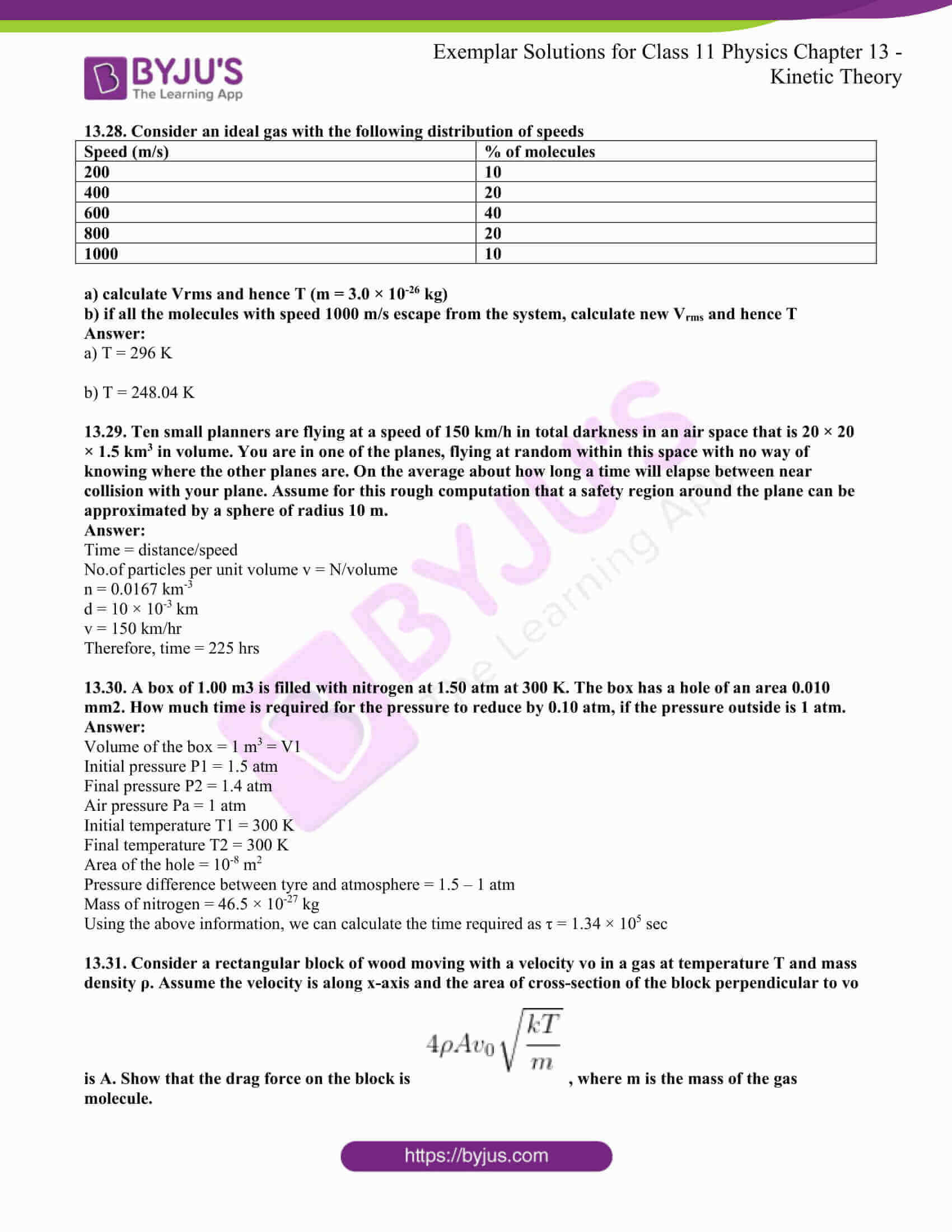### Multiple-choice Questions I

13.1. A cubic vessel (with faces horizontal + vertical) contains an ideal gas at NTP. The vessel is being carried by a rocket which is moving at a speed of 500 m/s in the vertical direction. The pressure of the gas inside the vessel as observed by us on the ground

a) remains the same because 500 m/s is very much smaller than vrms of the gas

b) remains the same because the motion of the vessel as a whole does not affect the relative motion of the gas molecules and the walls

c) will increase by a factor equal to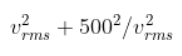where vrms was the original mean square velocity of the gas

d) will be the difference on the top wall and bottom wall of the vessel

The correct answer is b) remains the same because the motion of the vessel as a whole does not affect the relative motion of the gas molecules and the walls

13.2. 1 mole of an ideal gas is contained in a cubical volume V, ABCDEFGH at 300 K. One face of the cube (EFGH) is made up of a material which totally absorbs any gas molecule incident on it. At any given time,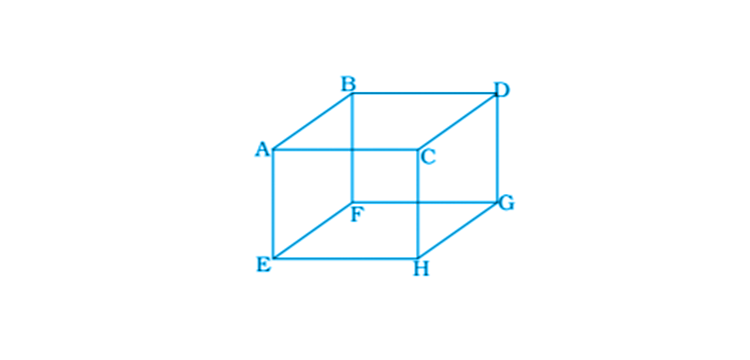a) the pressure on EFGH would be zero

b) the pressure on all the faces will the equal

c) the pressure of EFGH would be double the pressure on ABCD

d) the pressure on EFGH would be half that on ABCD

The correct answer is d) the pressure on EFGH would be half that on ABCD

13.3. Boyle’s law is applicable for an

b) isothermal process

c) isobaric process

d) isochoric process

The correct answer is b) isothermal process

13.4. A cylinder containing an ideal gas is in a vertical position and has a piston of mass M that is able to move up or down without friction. If the temperature is increased,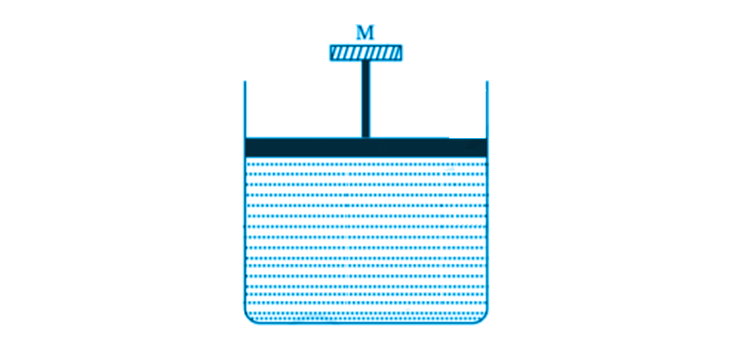a) both p and V of the gas will change

b) only p will increase according to Charle’s law

c) V will change but not p

d) p will change but not V

The correct answer is c) V will change but not p

13.5. Volume versus temperature graphs for a given mass of an ideal gas are shown in the figure at two different values of constant pressure. What can be inferred about the relation between P1 and P2?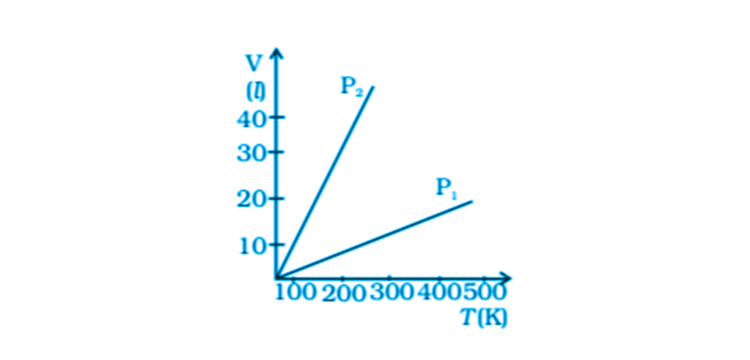a) P1 > P2

b) P1 = P2

c) P1 < P2

d) data is insufficient

The correct answer is a) P1 > P2

13.6. 1 mole of H2 gas is contained in a box of volume V = 1.00 m3 at T = 300 K. The gas is heated to a temperature of T = 3000 K and the gas gets converted to a gas of hydrogen atoms. The final pressure would be

a) same as the pressure initially

b) 2 times the pressure initially

c) 10 times the pressure initially

d) 20 times the pressure initially

The correct answer is d) 20 times the pressure initially

13.7. A vessel of volume V contains a mixture of 1 mole of hydrogen and 1 mole of oxygen. Let f1(v)dv, denote the fraction of molecules with speed between v and (v + dv) with f2(v)dv similarly for oxygen. Then

a) f1(v) + f2(v) = f(v) obeys the Maxwell’s distribution law

b) f1(v), f2(v) will obey the Maxwell’s distribution law separately

c) neither f1(v) nor f2(v) will obey the Maxwell’s distribution law

d) f2(v) and f1(v) will be the same

The correct answer is b) f1(v), f2(v) will obey the Maxwell’s distribution law separately

13.8. An inflated rubber balloon contains one mole of an ideal gas, has a pressure p, volume V, and temperature T. If the temperature rises to 1.1T and the volume is increased to 1.05V, the final pressure will be

a) 1.1 p

b) p

c) less than p

d) between p and 1.1

The correct answer is d) between p and 1.1

### Multiple Choice Questions II

13.9. ABCDEFGH is a hollow cube made of an insulator. Face ABCD has a positive charge on it. Inside the cube, we have ionized hydrogen. The usual kinetic theory expression for pressure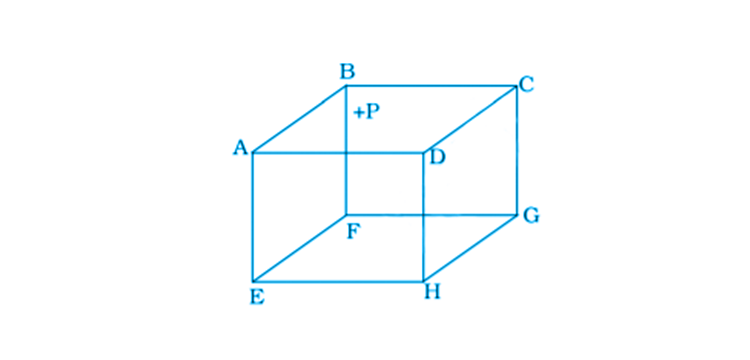a) will be valid

b) will not be valid since the ions would experience forces other than due to collision with the walls

c) will not be valid since collisions with walls would not be elastic

d) will not be valid because isotropy is lost

b) will not be valid since the ions would experience forces other than due to collision with the walls

d) will not be valid because isotropy is lost

13.10. Diatomic molecules like hydrogen have energies due to both translational as well as rotational motion. From the equation in kinetic theory pV = 2/3 E, E is

a) the total energy per unit volume

d) only the translational part of energy because rotational energy is very small compared to the translational energy

c) only the translational part of the energy because during collisions with the wall pressure relates to change in linear momentum

d) the translational part of the energy, because rotational energies of molecules can be either sign and its average over all the molecules is zero

The correct answer is c) only the translational part of the energy because during collisions with the wall, pressure relates to a change in linear momentum

13.11. Ina diatomic molecules, the rotational energy at a given temperature

a) obeys Maxwell’s distribution

b) have the same value for all molecules

c) equals the translational kinetic energy for each molecule

d) is 2/3rd the translational kinetic energy for each molecule

a) obeys Maxwell’s distribution

d) is 2/3rd the translational kinetic energy for each molecule

13.12. Which of the following diagrams depicts ideal gas behaviour?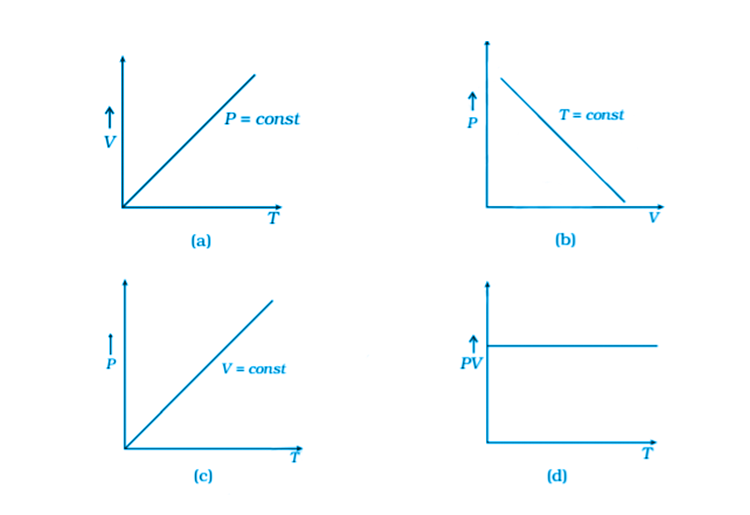a)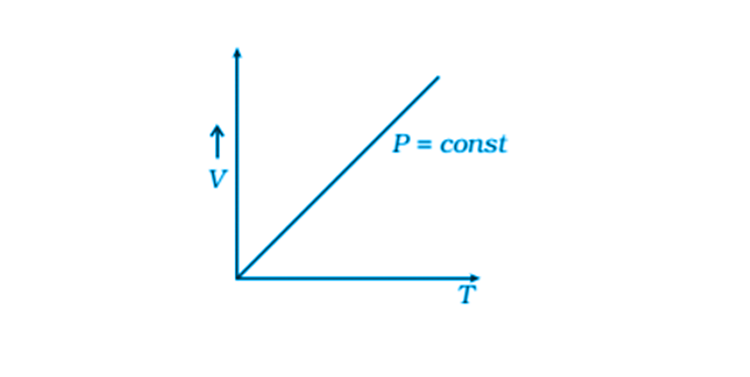c)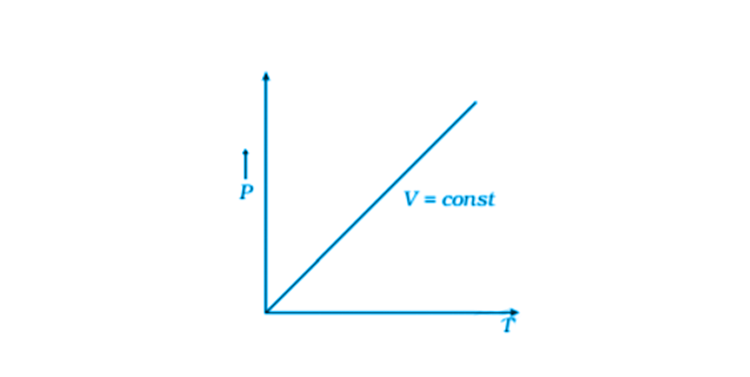13.13. When an ideal gas is compressed adiabatically, its temperature rises: the molecules on the average have more kinetic energy than before. The kinetic energy increases,

a) because of collisions with moving parts of the wall only

b) because of collisions with the entire wall

c) because the molecules gets accelerated in their motion inside the volume

d) because of the redistribution of energy amongst the molecules

The correct answer is a) because of collisions with moving parts of the wall only

13.14. Calculate the number of atoms in 39.4 g gold. The molar mass of gold is 197 g/mole.

Molar mass = 6.023 × 1023 atoms

Mass of gold, m = 39.4 g

Molar mass of gold, M = 197 g/mol

196 g of gold contains 6.023 × 1023 atoms

39.4 g of gold contains 1.20 × 1023 atoms.

13.15. The volume of a given mass of a gas at 27oC, 1 atm is 100 cc. What will be its volume at 327oC?

T1 = 27oC

T1 = 300 K

V1 = 100 cm3

We know that V is proportional to T

V/T = constant

V1/T1 = V2/T2

V2 = V1(T2/T1)

V2 = 200 cc

13.16. The molecules of a given mass of gas have root mean square speeds of 100 m/s at 27oC and 1.00 atmospheric pressure. What will be the root mean square speeds of the molecules of the gas at 127oC and 2.0 atmospheric pressure?

Vrms = 100 m/s

T1 = 300 K

T2 = 400 K

Vrms = √3RT/M

V2rms =?

Vrms = 115.4 m/s

13.17. Two molecules of a gas have speeds of 9 × 106 m/s and 1 × 106 m/s, respectively. What is the root mean square speed of these molecules?

V1 = 9 × 106 m/s

V2 = 1 × 106 m/s

Vrms = 6.4 × 106 m/s

13.18. A gas mixture consists of 2.0 moles of oxygen and 4.0 moles of neon a temperature T. Neglecting all vibrational modes, calculate the total internal energy of the system. (Oxygen has two rotational modes.)

Degree of freedom of oxygen = 5

Total internal energy of 2-mole oxygen = 5RT

Total internal energy of 4 mole of Ne = 6RT

The total internal energy of the system = 11 RT

13.19. Calculate the ratio of the mean free paths of the molecules of two gases having molecular diameters 1 A and 2 A. The gases may be considered under identical conditions of temperature, pressure, and volume.

The ratio of the mean free paths of the molecules is 4:1.

13.20. The container shown in the figure has two chambers, separated by a partition, of volumes V1 = 2.0 litre and V2 = 3.0 litre. The chambers contain μ1 = 4.0 and μ2 = 5.0 moles of a gas at pressure p1 = 1.00 atm and p2 = 2.00 atm. Calculate the pressure after the partition is removed and the mixture attains equilibrium.

 V1 V2 μ1 μ2 p1 p2

For an ideal gas, PV = μRT

P1V1 = μ1R1T1

P2V2 = μ2R2T2

P1 = 1 atm

P2 = 2 atm

V1 = 2L

V2 = 3L

T1 = T = T2

μ1 = 4

μ2 = 5

Substituting all the values we get, P = 1.6 atm

13.21. A gas mixture consists of molecules of tyres A, B, and C with masses mA > mB > mC. Rank the three types of molecules in decreasing order of

a) average KE

b) rms speeds

a) Pressure and temperature are the same, therefore,

KEc > KEb > KEa

b) When P and T are constant, (Vrms)c > (Vrms)b > (Vrms)a

13.22. We have 0.5 g of hydrogen gas in a cubic chamber of size 3 cm kept at NTP. The gas in the chamber is compressed, keeping the temperature constant till a final pressure of 100 atm. Is one justified in assuming the ideal gas law, in the final state?

Volume of 1 molecule = 4/3 πr3 = 4.20 × 10-30 m3

No.of moles in 0.5 g H2 gas = 0.25 mole

Volume of H2 molecule in 0.25 mole = 1.04×6.023× 10+23-30

13.23. When air is pumped into a cycle tyre, the volume and pressure of the air in the tyre both are increased. What about Boyle’s law in this case?

PV = P(m/ρ) = constant

P/ ρ = constant

Volume = m/ ρ where m is constant

Therefore, when the air is pumped into the tyre of the cycle, the mass of air increases as the no.of molecules increases. Therefore, Boyle’s law is only applicable when the mass of the gas remains fixed.

13.24. A balloon has 5.0 g mole of helium at 7oC. Calculate

a) the number of atoms of helium in the balloon

b) the total internal energy of the system

Average KE per molecule = 3/2kT

No.of moles of helium, n = 5 g mole

T = 280 K

a) No.of atoms of helium in the balloon = 30.015 × 1023

b) KE = 3/2 kbT

Total internal energy = 1.7 × 104J

13.25. Calculate the number of degrees of freedom of molecules of hydrogen in 1 cc of hydrogen gas at NTP.

The volume occupied by the molecules of gas = 22400 cc

No.of molecules in 1 cc of hydrogen = 2.688 × 1019

Hydrogen has a total of 5 degrees of free as it is a diatomic gas

Therefore, the total degrees of freedom = 1.344 × 1020

13.26. An insulated container containing monoatomic gas of molar mass m is moving with a velocity vo. If the container is suddenly stopped, find the change in temperature.

The final KE of the gas = 0

Change in KE, ∆K= 1/2 (nm)v2

∆T is the change in the temperature

∆U = nCv∆T

∆K = ∆U

∆T = mv02/3R

13.27. Explain why

a) there is no atmosphere on moon

b) there is a fall in temperature with altitude

a) There is no atmosphere on the moon because the gravitational force is small, and the Vrms is greater on the moon such that the escape velocity of the molecule is greater than that of the air. Also, the distance between the moon and the sun is the same as the distance between the moon and the earth. Therefore, the RMS speed of the molecule increases such that it can be more than the escape velocity. Therefore, there is no atmosphere on the moon.

b) There is a fall in temperature with altitude because at the higher altitude, the gas molecules expand, and there is a decrease in temperature as the expansion of gas results in cooling of the surrounding. This results in an increase in the kinetic energy of the molecules. When the kinetic energy increases, temperature decreases.

13.28. Consider an ideal gas with the following distribution of speeds

 Speed (m/s) % of molecules 200 10 400 20 600 40 800 20 1000 10

a) calculate Vrms and hence T (m = 3.0 × 10-26 kg)

b) if all the molecules with speed 1000 m/s escape from the system, calculate new Vrms and hence T

a) T = 296 K

b) T = 248.04 K

13.29. Ten small planners are flying at a speed of 150 km/h in total darkness in an air space that is 20 × 20 × 1.5 km3 in volume. You are in one of the planes, flying at random within this space with no way of knowing where the other planes are. On average about how long a time will elapse between near collision with your plane? Assume for this rough computation that a safety region around the plane can be approximated by a sphere of radius 10 m.

Time = distance/speed

No.of particles per unit volume v = N/volume

n = 0.0167 km-3

d = 10 × 10-3 km

v = 150 km/hr

Therefore, time = 225 hrs

13.30. A box of 1.00 m3 is filled with nitrogen at 1.50 atm at 300 K. The box has a hole of an area 0.010 mm2. How much time is required for the pressure to reduce by 0.10 atm, if the pressure outside is 1 atm?

Volume of the box = 1 m3 = V1

Initial pressure P1 = 1.5 atm

Final pressure P2 = 1.4 atm

Air pressure Pa = 1 atm

Initial temperature T1 = 300 K

Final temperature T2 = 300 K

Area of the hole = 10-8 m2

Pressure difference between tyre and atmosphere = 1.5 – 1 atm

Mass of nitrogen = 46.5 × 10-27 kg

Using the above information, we can calculate the time required as τ = 1.34 × 105 sec

13.31. Consider a rectangular block of wood moving with a velocity vo in a gas at temperature T and mass density ρ. Assume the velocity is along the x-axis and the area of the cross-section of the block perpendicular to vo is A. Show that the drag force on the block is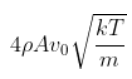, where m is the mass of the gas molecule.

Let ρm is the no.of molecules per unit volume

Change in momentum by a molecule on front side = 2m (v + v0)

Change in momentum by a molecule on backside = 2m (v – v0)

No.of molecules striking front side = 1/2 [A(v+v0)∆t] ρm

No.of molecules striking back side = 1/2 [A(v-v0)∆t] ρm

Solving the above equation by considering the KE of the gas molecule, we get the dragging force as 4m A ρmv0√kgT/m

Some of the different macroscopic properties of gases, which can be explained with the help of kinetic theory are, listed below:

1. Pressure
2. Temperature
3. Viscosity
4. Thermal Conductivity
5. Volume

#### Important Topics Covered in Chapter 13 Kinetic Theory

1. Molecular nature of matter
2. Behaviour of gases
3. Kinetic theory of an ideal gas
4. Law of equipartition of energy
5. Specific heat capacity
6. Mean free path

### Why Opt for BYJU’S?

BYJU’S provides the best study materials for CBSE Class 11 Physics. Apart from the study materials, we also provide students with the best support from the country’s top teachers to clear their doubts about all topics and subjects. BYJU’S is committed to giving quality education to students, thus helping  them score good marks in the final examinations.